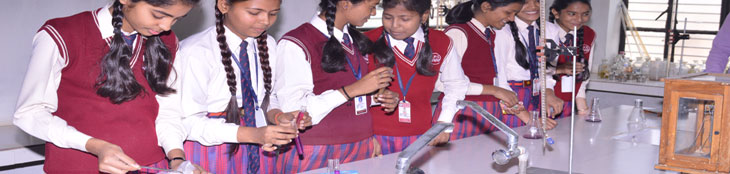# Maths LabMathematics is a subject which requires single minded concentration and continuous efforts to achieve perfect and accurate learning. Mathematics has to be learnt by doing rather than by learning.With this aim various activities are performed to help exploration of mathematical facts through experimentation.

• Various geometric properties are verified through paper cutting and paper folding.

• Three dimensional models are used to explain some abstract concepts.

• Factorizations of algebraic expressions are explained through paper cutting and folding.

• Verification of Pythagoras theorem through working model.

• To find the probability using colored pearls and playing cards.

• Different 3-D models are made with the help of unit cubes.

• Making the charts of different formulae.

• Portraits of mathematicians and their contributions - charts are made by students.

Geo boards are used for graphical presentation of linear equations ,their consistency and to draw various polygons